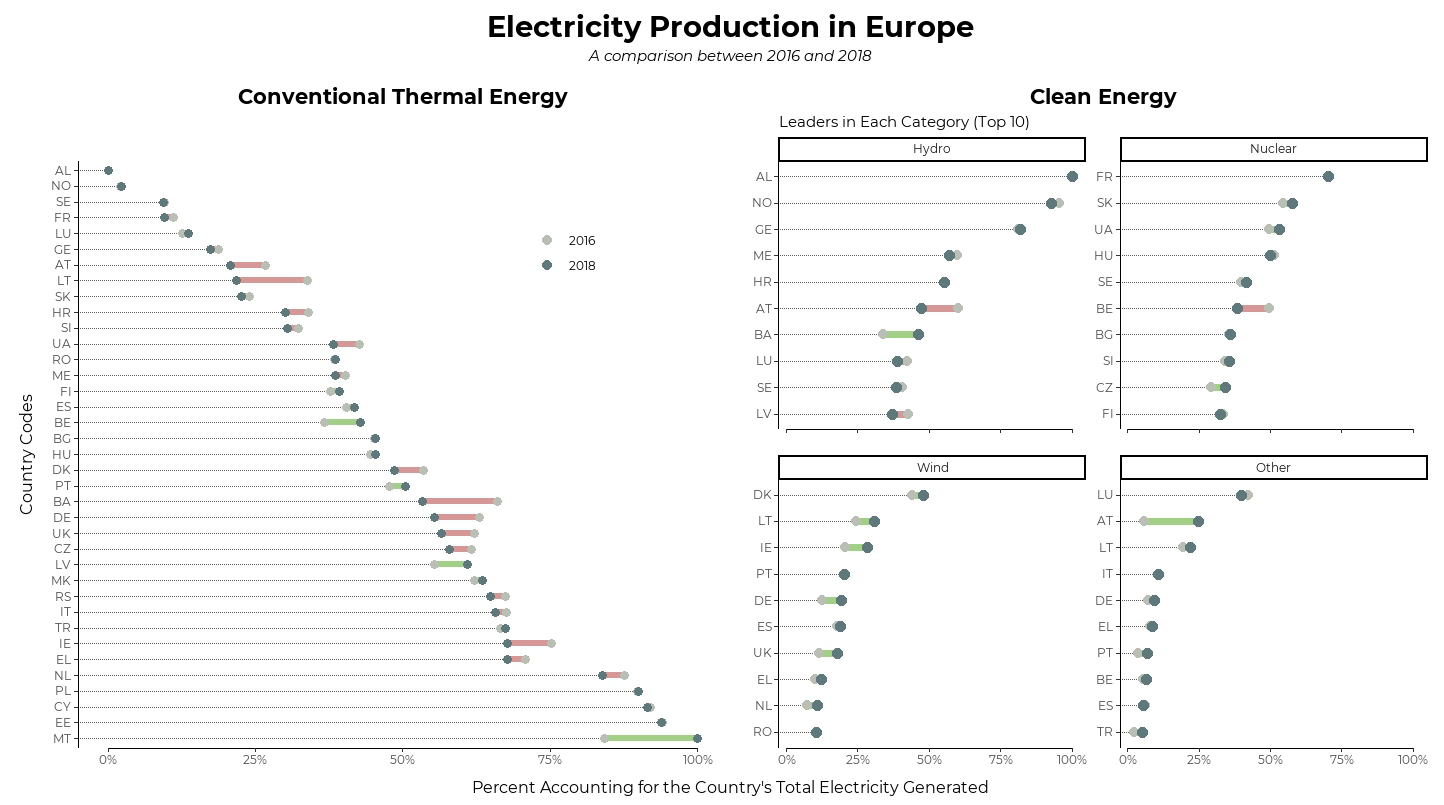# TidyTuesday 2020 Week 32

A dumbbell chart visualization of energy production trends among European countries

June Choe (University of Pennsylvania Linguistics)https://live-sas-www-ling.pantheon.sas.upenn.edu/
08-04-2020

## Visualization### Things I learned

• `geom_dumbbell()` from `{ggalt}`

• `coord_capped_cart()` and `facet_rep_wrap()` from `{lemon}`

• Using the `reorder_within()` + `facet_wrap(scales = "free_y")` + `scale_y_reordered()` combo to sort within facets.

• Using `override.aes` argument to manipulate legend aesthetics after they’re generated by the `geom_*()`s

• Using `slice_max()` instead of `top_n()` to catch up with the new `{dplyr}` update

### Things to improve

• Font sizing and image resolution

• Placement and size of legend is sorta awkward.

• Plot feels too… empty. I think I treated this too much like a figure for a journal article. Maybe add some background color next time?

## Code

Also available on github

``````library(tidyverse)
library(tidytuesdayR)
library(lemon)
library(ggalt)
library(patchwork)
library(extrafont)

theme_set(theme_classic())

### Data

energy_types <- tuesdata\$energy_types

energy_types_tidy <- energy_types %>%
pivot_longer(where(is.double), names_to = "Year", values_to = "GWh")

plot_data <- energy_types_tidy %>%
add_count(country, Year, wt = GWh, name = "Total") %>%
mutate(GWh_prop = GWh/Total) %>%
select(-country_name, -GWh, -Total , -level) %>%
filter(Year %in% c(2016, 2018))

### Plotting

p1 <- plot_data %>%
filter(type == "Conventional thermal") %>%
pivot_wider(names_from = Year, values_from = GWh_prop) %>%
mutate(country = fct_reorder(country, `2018`, max, .desc = TRUE)) %>%
mutate(increase = (`2018` - `2016`) > 0) %>%
ggplot() +
geom_dumbbell(
aes(y = country, x = `2016`, xend = `2018`, color = increase),
dot_guide = TRUE, dot_guide_size = 0.25,
size = 2, colour_x = "#babfb6", colour_xend = "#5f787b"
) +
scale_color_manual(values = c("#d69896", "#a1cf86"), labels = c("2016", "2018")) +
guides(color = guide_legend(override.aes = list(color = c("#babfb6", "#5f787b"), size = 3))) +
labs(title = "Conventional Thermal Energy",
y = "Country Codes",
color = NULL) +
theme(legend.position = c(.75, .85),
axis.title.y = element_text(size = 12, vjust = 5))

p2 <- plot_data %>%
filter(type != "Conventional thermal") %>%
pivot_wider(names_from = Year, values_from = GWh_prop) %>%
mutate(type = fct_lump(type, n = 3, w = `2018`)) %>%
group_by(type, country) %>%
summarize(`2016` = sum(`2016`), `2018` = sum(`2018`)) %>%
slice_max(`2018`, n = 10, with_ties = FALSE) %>%
mutate(country = tidytext::reorder_within(country, `2018`, type)) %>%
mutate(increase = (`2018` - `2016`) > 0) %>%
ggplot() +
geom_dumbbell(
aes(y = country, x = `2016`, xend = `2018`, color = increase),
dot_guide = TRUE, dot_guide_size = .4,
size = 2.5, colour_x = "#babfb6", colour_xend = "#5f787b",
show.legend = FALSE
) +
scale_color_manual(values = c("#d69896", "#a1cf86")) +
tidytext::scale_y_reordered() +
facet_rep_wrap(~type, scales = "free_y") +
labs(title = "Clean Energy",
subtitle = "Leaders in Each Category (Top 10)",
y = NULL)

patched <- p1 + p2 &
coord_capped_cart(bottom = "both") &
scale_x_continuous(labels = scales::percent) &
labs(x = NULL) &
theme(
plot.title = element_text(hjust = 0.5, size = 16, face = "bold"),
text = element_text(family = "Montserrat"),
panel.grid.major.y = element_blank(),
plot.margin = unit(c(.4,.2,.2,.4), "cm"),
plot.background = element_rect(color = "transparent")
)

patched + plot_annotation(title = "Electricity Production in Europe",
subtitle = "A comparison between 2016 and 2018",
caption = "Percent Accounting for the Country's Total Electricity Generated",
theme = list(plot.title = element_text(size = 22),
plot.subtitle = element_text(face = "italic", hjust = .5),
plot.caption = element_text(size = 12, hjust = .5)))
``````